# How to extract word from string in Excel: first, last, Nth, and more

The tutorial demonstrates a quick, precise, and error-free method to extract words in Excel without lengthy and complex formulas, thanks to a custom function.

Have you ever faced the challenge of extracting specific words from text within an Excel cell? Getting the first or last word can be relatively easy using built-in Excel functions. However, extracting an arbitrary word from the middle of the text can be much more complex. In this article, we will explain how to efficiently and accurately accomplish the task using a custom function.

## Custom function to extract word from string in Excel

In one of the previous articles, we discussed how to extract the first, last, or Nth word from a cell using a MID formula. Now, we'll show you how to replace all those lengthy formulas with just one user-defined function. This will save you a lot of time and prevent inadvertent errors when crafting formulas.

The custom ExtractWord function presented in this article will enable you to:

• Extract the first or last word from a cell.
• Pull the second, third, or any specific word from a string.
• Utilize any word delimiters such as a space, comma or semicolon.
• Get any word containing a specified character from the text.

To begin, add the following code to your workbook as described in How to insert VBA code in Excel.

Tip. If you plan to use this function frequently, we recommend storing it in an add-in file rather than your current workbook for better accessibility. You can find detailed instructions on how to do this here: Using add-ins to store custom functions.

Once the function's code is added to your Excel, you can use it just like any other native function. If you're new to UDFs and want to learn more, you can find full details in How to use custom functions in Excel.

### The function’s syntax

The syntax for using the custom function is as follows:

ExtractWord(data_range, [word_num], [delimiter], [char])

Required argument:

• Data_range – This is the cell or range of cells from which you want to extract a word.

Optional arguments:

• Word_num – This argument specifies the ordinal number of the word you want to extract:
• Positive number – pulls a word from the beginning of the string. For example, to extract the first word, input 1 or leave empty.
• Negative number – pulls a word from the end of the string. For instance, to extract the last word, input -1.
• Defaults to 1 - if the argument is omitted, the function will extract the first word.
• Delimiter – Use this argument to specify the word delimiter. If not specified, it is assumed that words are separated by spaces. The delimiter is case-sensitive. Consecutive delimiters are treated as a single delimiter.
• Char – Use this argument when you want to extract a word containing a specific character. If you also set the word_num argument, the function will extract the first, second, third, …, or last word containing the specified character.

With the syntax established let’s move on to practical examples and take a closer look at how to use this powerful function in your Excel spreadsheets.

## How to extract first word in Excel

Let's start with the most common scenario - extracting the first word from an Excel cell. Assuming the target text is in cell A3, the custom function is as straightforward as it gets:

`=ExtractWord(A3, 1)`

In fact, you can make it even shorter by omitting the second argument as word_num defaults to 1:

`=ExtractWord(A3)`

Now, let's compare this to the traditional MID formula for extracting first word from string:

`=MID(A3, 1, SEARCH(" ", A3) - 1)`

As you can see, the results are the same, but the custom function is more concise and user-friendly.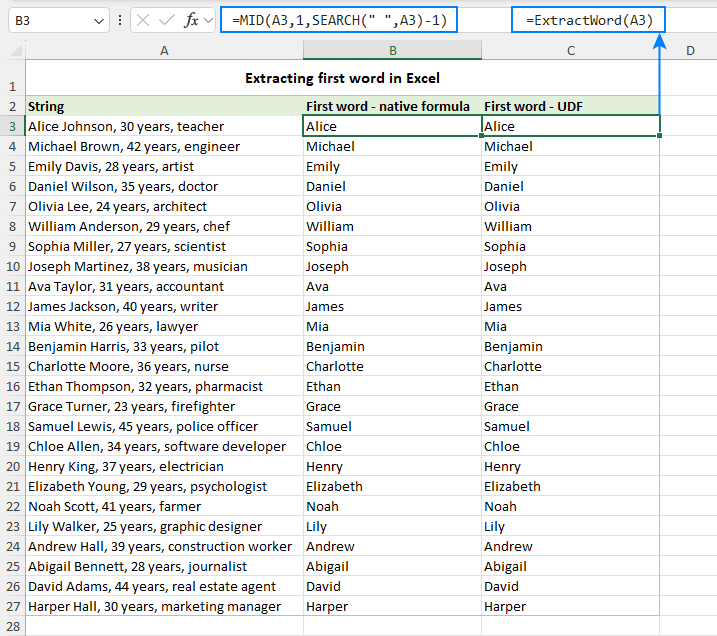## How to extract last word in Excel

To extract the last word from a text string in Excel using the custom function, set the word_num argument to -1. Here's how the formula looks:

`=ExtractWord(A3, -1)`

Now, if you were to attempt the same task using native Excel functions, you'd need to create a much longer and more complex formula that combines six different functions. Here's what it would look like:

`=TRIM(RIGHT(SUBSTITUTE(A3, " ", REPT(" ", LEN(A3))), LEN(A3)))`

Despite the complexity of the native formula, the results are absolutely identical to what you get with our custom function: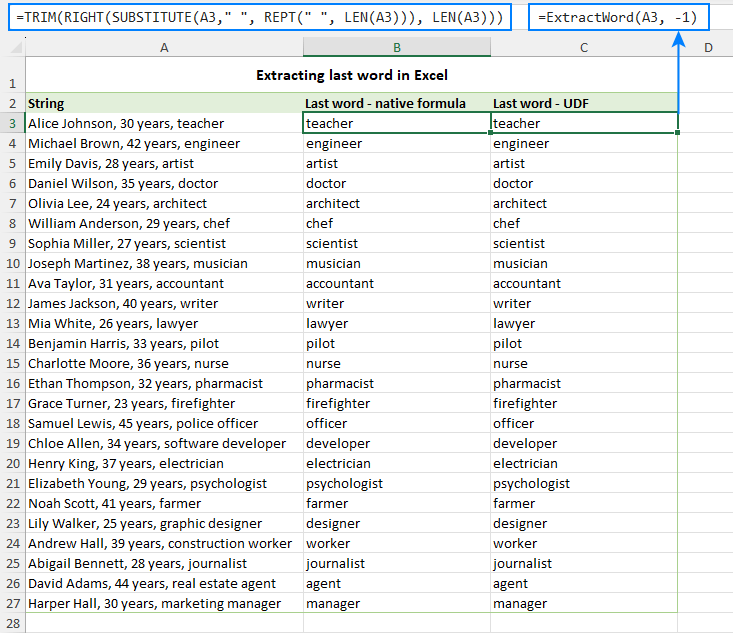By opting for the custom function, you not only simplify your Excel formulas but also enhance the clarity and readability of your spreadsheet.

## How to get Nth word from Excel cell

To extract any specific word from a text string, you just need to tell the ExtractWord function which word you want.

Let's say you wish to extract the second word from cell A3. Here's the formula:

`=ExtractWord(A3, 2)`

If your goal is the second word from the end of the string (the last but one word), the formula changes to:

`=ExtractWord(A3, -2)`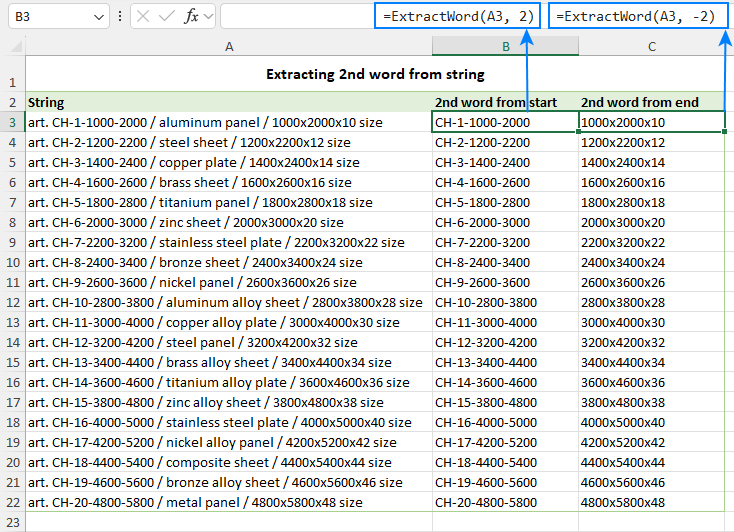Also, you can use a more flexible approach by putting the word number in another cell. For example, with the target word number in cell B3, the formula takes this form:

`=ExtractWord(A3, B3)`

This formula is much shorter and simpler than one using regular Excel functions, but it gives you the same result:

`=TRIM(MID(SUBSTITUTE(TRIM(\$A\$3), " ", REPT(" ", LEN(\$A\$3))), (B3-1)*LEN(\$A\$3)+1, LEN(\$A\$3)))`

For the detailed explanation of this formula, see MID function to extract Nth word from text.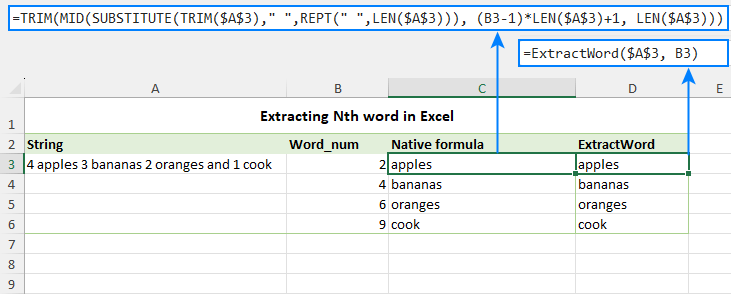By using this method, you save a lot of time. Plus, it's hard to make mistakes with the compact custom function, whereas longer formulas might lead to accidental errors.

## Extracting words with custom delimiter

In all the previous examples, we focused on extracting words separated by spaces. But that's not always the case. When you import data into Excel from other programs, they can use various symbols as separators such as semicolons, slashes, vertical bars, and more. In such situations, the third argument in the ExtractWord function comes in handy.

Let's illustrate this with an example. Suppose you want to get the second word from cell A3, where words are delimited by " / " (space-slash-space). The formula looks like this:

`=ExtractWord(A3, 2, " / ")`

It's important to note that we include a space character on both sides of the slash symbol to avoid capturing leading and trailing spaces in the results. If your source records use slashes without spaces, then you'd simply use "/" for the delimiter argument.

As shown in the screenshot below, in this case, you can even get multiple words as a result. This happens because the space here is treated as a regular character, not a separator. In other words, any and all characters between the delimiters specified in the formula are considered a single word.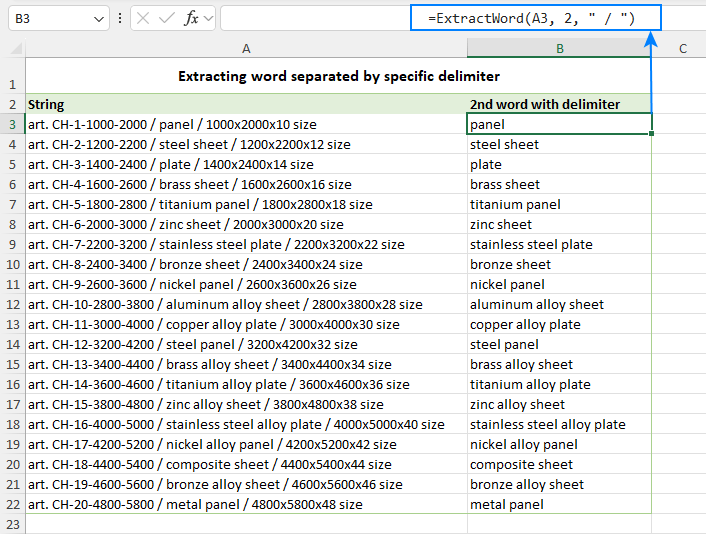Note. The delimiter argument is case-sensitive, which may be crucial when using a letter as a separator, as uppercase and lowercase letters are treated as different characters.

## How to get word containing certain character

To extract a word containing a particular character, you can utilize the fourth argument of the ExtractWord function, aptly named char. This argument defines which specific character you want to find within text and instructs the function to extract the word containing that character.

For instance, financial documents created in Excel often contain various currency amounts within text strings. Instead of using complex regular expressions to extract these figures, you can employ a straightforward custom function.

`=ExtractWord(A3, 1, , "\$")`

In this scenario, the formula returns the first word containing the symbol "\$" from the text in A3.

The example below illustrates how you can use a reference to the cell containing the target character (B3). You can also specify which occurrence of the word with the desired character to return (cells A6:A9). As shown, there are four words with the "\$" symbol in the source string. You can retrieve any of them by entering the following formula in cell B6 and copying it down through B9:

`=ExtractWord(\$A\$3, A6, , \$B\$3)`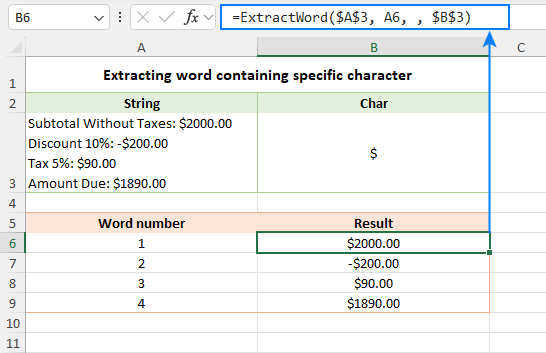## How to extract word containing specific text

In addition to characters, the ExtractWord function excels at extracting words that contain certain text. To achieve this, simply provide the target text (substring) as the char argument.

For example, to extract email addresses with the "gmail" domain, the formula is as follows:

`=ExtractWord(A3, , , "gmail")`

The result is displayed in the screenshot below: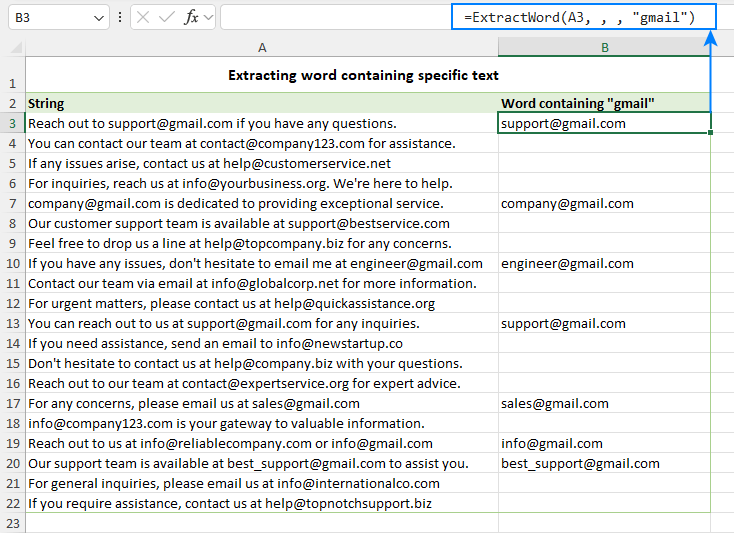## How to extract text after word in Excel

To extract text that comes after a specific word, you need to do two things:

• Provide the target word as the delimiter argument.
• Supply 2 for the word_number argument to indicate that you want to retrieve the word after the delimiter.

Yep, it’s that simple!

For instance, to pull the text following the phrase "Error code", the formula looks like this:

`=ExtractWord(A3, 2, "Error code")`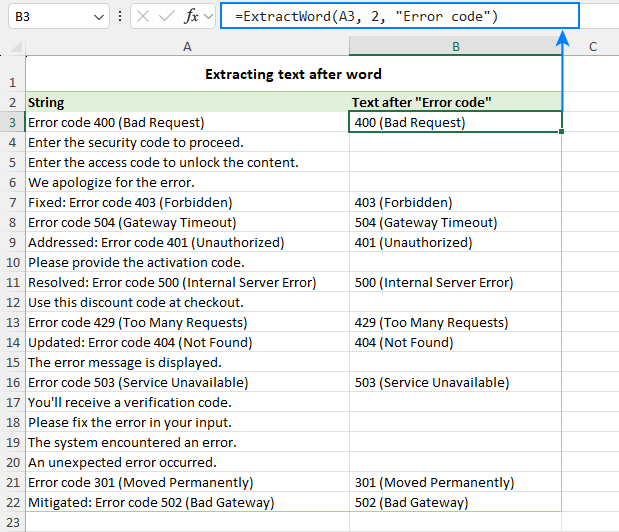Note. Please remember that the delimiter is case-sensitive, so using "error code" and "Error code" will yield different results. Make sure to match the case of your target word accurately when using this method.

## How to split text into words by any delimiter

This particular use of the ExtractWord function might not be immediately apparent, but it's a powerful technique. By incorporating the ROW or COLUMN function within the ExtractWord formula, you can effectively split a text string into separate cells either vertically or horizontally.

### Split string in rows

To split the text in A3 into rows using " / " as the delimiter, the formula is:

`=ExtractWord(\$A\$3, ROW(A1), " / ")`

Place this formula in cell B3, then copy it down the column. You'll achieve the following result: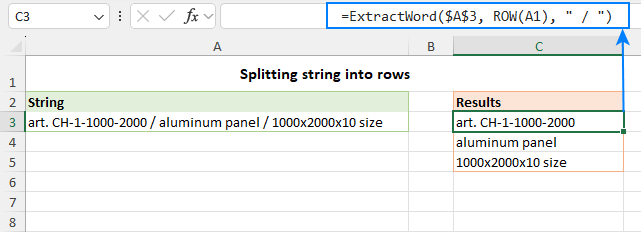As you copy the formula down the column, the relative reference to cell A1 changes to A2, A3, and so on. As a result, the ROW(A1) function returns 1, ROW(A2) returns 2, and so forth. This allows you to sequentially extract the first, second, and subsequent words into separate cells in a given column.

### Split string in columns

To place the words into separate columns, use the COLUMN function instead of ROW to generate numbers for the word_num argument:

`=ExtractWord(\$A\$3, COLUMN(A1), " / ")`

Enter the formula in the first cell (A8), and then copy it across the row. The original cell content will be split into individual columns accordingly.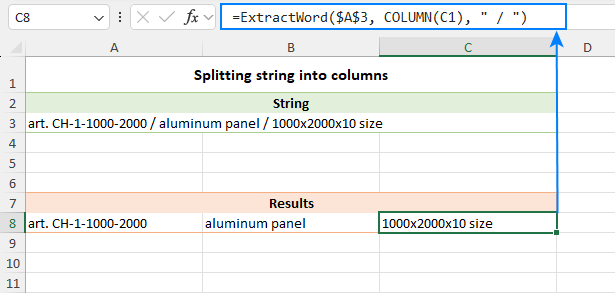In Excel 365, you can achieve a similar result using the TEXTSPLIT function. However, in earlier versions where TEXTSPLIT is unavailable, this technique can serve as a reliable alternative to Excel's cell splitting tools or string splitting formulas.

## How to get a word from a range of cells

The ExtractWord function isn't limited to extracting words from individual cells; it can also extract words from a range. To do this, specify a cell range as the first argument. Here's an example:

`=ExtractWord(\$A\$3:\$A\$13, C3)`

The screenshot below shows how you can retrieve any word from a range using word numbers specified in predefined cells (C3:C12 in our case).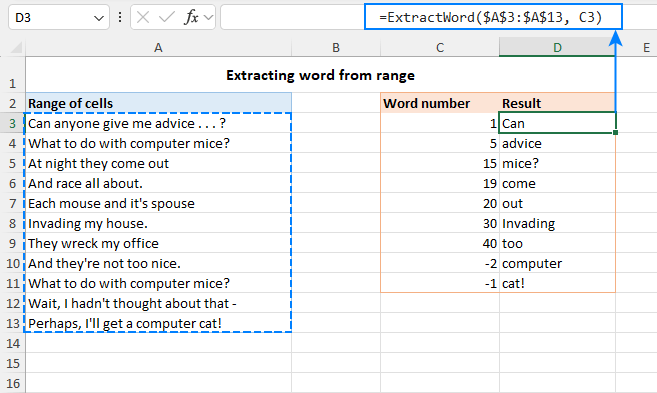Tip. When extracting words from a range, you can define your own delimiter. Additionally, you can specify a character that the word should contain. In other words, in all the examples we've covered earlier, you can supply a range instead of an individual cell.

This is how to extract words from Excel cells using a custom function. Whether you want to grab specific words or find content based on certain characters, this handy tool will help you discover fresh insights. So, the next time you're dealing with text in Excel, keep the ExtractWord function in mind - it can be the secret to improving your data tasks and achieving better results.